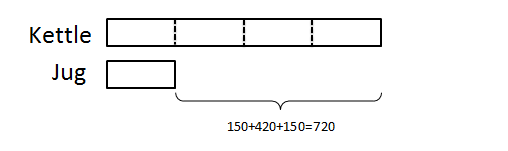# The #1 Key to Solving Problem Sums…that Most Parents Ignore

Problem sums, or math word problems, are one of the most challenging learning tasks for primary school students. Parents often worry about their children’s ability to handle these complex math questions, which require advanced thinking and special methods to solve. As such, kids are frequently drilled on problem sums by parents or even sent for special math tuition classes and workshops to learn various special problem sums solving techniques. Unfortunately, all of these fail to address the number one key to solving problem sums, which is to understand the question.## The #1 Key to Solving Problem Sums: Comprehension

Mathematical inability is usually the last reason why children are unable to solve problem sums and math word problems. In fact, problem sums require only basic arithmetic skills like addition, subtraction, multiplication, division, fractions, and ratios. These questions are designed, rather, to test students’ understanding and application of mathematical concepts.

If your child does not comprehend the question and merely uses a certain technique to solve a problem sum, he or she will have even more trouble with Math and other subjects later on in their learning journey.

Before trying various heuristics to solve a problem sum, ensure that your child comprehends the question first:

1. Read through the entire question
2. Break the question down into parts
3. Determine the order of steps to get to the solution

Once the order of steps has been determined, your child can then apply methods such as model drawing or supposition to solve the problem sum.

#### Example Question (Primary 3)

A kettle has 420ml more water than a jug. When 150ml of water is poured from the jug into the kettle, there is 4 times as much water in the kettle as the jug. How much water was there in the jug at first?

Here is how the problem can be broken down into parts:

• The kettle has 420ml more water than the jug.• When 150ml of water is poured from the jug into the kettle,• There is 4 times as much water in the kettle as the jug.With the problem sum broken down into parts and visualized using models, your child can then solve the problem using basic arithmetic.

## How to Practise Problem Sums Comprehension

Instead of drilling your child on various techniques to solve different types of problem sums, focus on reading the question.

1. Get your child to go through various questions in his/her math workbook or assessment books without solving them.
2. Then, ask him/her to identify the questions which seem similar.
3. Determine the steps needed to get the solution, but don’t do the math. See if the steps can be applied to similar questions.

Increased reading is the key to improving your child’s English language comprehension skills. With better comprehension, you’ll find that your child can solve problem sums more easily and quickly.

Do you have any practical tips of your own (not “secret” techniques) for solving problem sums?